Next: Spontaneous Emission Up: Time-Dependent Perturbation Theory Previous: Absorption and Stimulated Emission

# Electric Dipole Approximation

In general, the wavelength of the type of electromagnetic radiation that induces, or is emitted during, transitions between different atomic energy levels is much larger than the typical size of a light atom. Thus,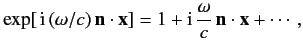(887)

can be approximated by its first term, unity (remember that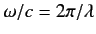). This approximation is known as the electric dipole approximation. It follows that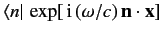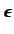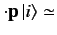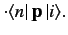(888)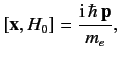(889)

so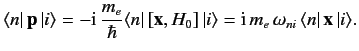(890)

Thus, making use of the electric dipole approximation, we obtain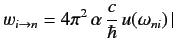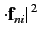(891)

for absorption, and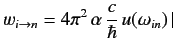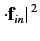(892)

for stimulated emission, where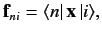(893)

and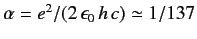is the fine structure constant.

Suppose that the radiation is polarized in the-direction, so that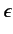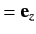. We have already seen, from Section 7.4, that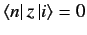unless the initial and final states satisfy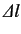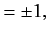(894)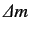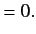(895)

Here,is the quantum number describing the total orbital angular momentum of the electron, and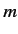is the quantum number describing the projection of the orbital angular momentum along the-axis. It is easily demonstrated that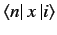and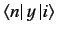are only non-zero if(896)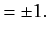(897)

Thus, for generally directed radiation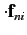is only non-zero if(898)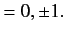(899)

These are termed the selection rules for electric dipole transitions. It is clear, for instance, that the electric dipole approximation allows a transition from a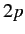state to a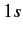state, but disallows a transition from a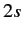to astate. The latter transition is called a forbidden transition.

Forbidden transitions are not strictly forbidden. Instead, they take place at a far lower rate than transitions that are allowed according to the electric dipole approximation. After electric dipole transitions, the next most likely type of transition is a magnetic dipole transition, which is due to the interaction between the electron spin and the oscillating magnetic field of the incident electromagnetic radiation. Magnetic dipole transitions are typically about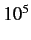times more unlikely than similar electric dipole transitions. The first-order term in Equation (887) yields so-called electric quadrupole transitions. These are typically about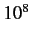times more unlikely than electric dipole transitions. Magnetic dipole and electric quadrupole transitions satisfy different selection rules than electric dipole transitions. For instance, the selection rules for electric quadrupole transitions are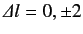. Thus, transitions that are forbidden as electric dipole transitions may well be allowed as magnetic dipole or electric quadrupole transitions.Next: Spontaneous Emission Up: Time-Dependent Perturbation Theory Previous: Absorption and Stimulated Emission
Richard Fitzpatrick 2013-04-08